# maxflow

## 语法

``mf = maxflow(G,s,t)``
``mf = maxflow(G,s,t,algorithm)``
``[mf,GF] = maxflow(___)``
``[mf,GF,cs,ct] = maxflow(___)``

## 说明

``mf = maxflow(G,s,t)` 返回节点 `s` 和 `t` 之间的最大流。如果图 `G` 未加权（即 `G.Edges` 不包含变量 `Weight`），则 `maxflow` 将所有图边的权重视为 1。`

``mf = maxflow(G,s,t,algorithm)` 指定要使用的最大流算法。此语法仅在 `G` 为有向图时可用。 `

``[mf,GF] = maxflow(___)` 支持上述语法中的任何输入参数，且可返回有向图对象 `GF`。`GF` 仅使用 `G` 中具有非零流值的边构造。`

``[mf,GF,cs,ct] = maxflow(___)` 还返回源和目标节点 ID `cs` 和 `ct`，表示与最大流相关联的最小割。`

## 示例

```s = [1 1 2 2 3 4 4 4 5 5]; t = [2 3 3 4 5 3 5 6 4 6]; weights = [0.77 0.44 0.67 0.75 0.89 0.90 2 0.76 1 1]; G = digraph(s,t,weights); plot(G,'EdgeLabel',G.Edges.Weight,'Layout','layered');```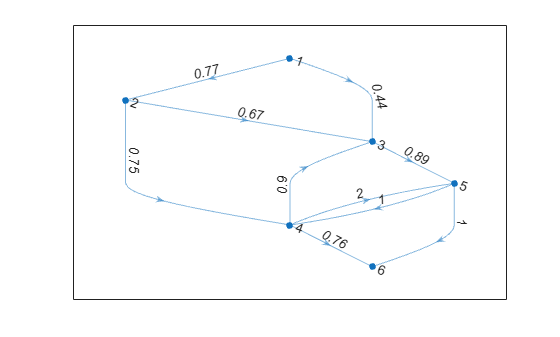`mf = maxflow(G,1,6)`
```mf = 1.2100 ```

```s = [1 1 2 2 3 3 4]; t = [2 3 3 4 4 5 5]; weights = [10 6 15 5 10 3 8]; G = digraph(s,t,weights); H = plot(G,'EdgeLabel',G.Edges.Weight);```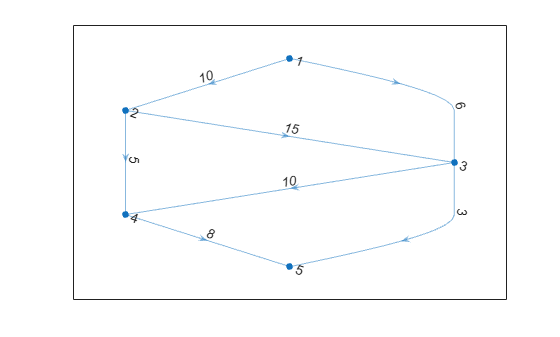`[mf,GF] = maxflow(G,1,5,'augmentpath')`
```mf = 11 ```
```GF = digraph with properties: Edges: [6x2 table] Nodes: [5x0 table] ```

```H.EdgeLabel = {}; highlight(H,GF,'EdgeColor','r','LineWidth',2); st = GF.Edges.EndNodes; labeledge(H,st(:,1),st(:,2),GF.Edges.Weight);```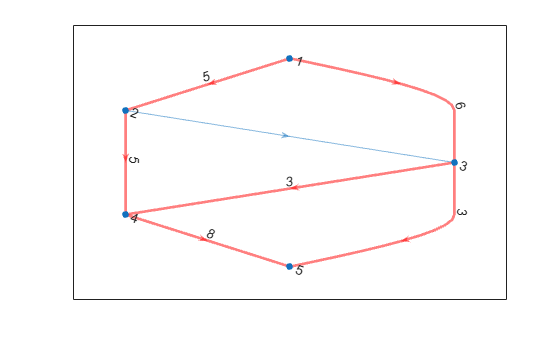```s = [1 1 2 3 3 4 4 5 5]; t = [2 3 3 2 5 5 6 4 6]; weights = [0.77 0.44 0.67 0.69 0.73 2 0.78 1 1]; G = digraph(s,t,weights); plot(G,'EdgeLabel',G.Edges.Weight,'Layout','layered')```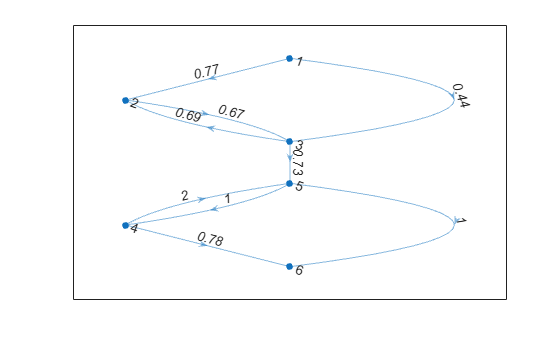`[mf,~,cs,ct] = maxflow(G,1,6)`
```mf = 0.7300 ```
```cs = 3×1 1 2 3 ```
```ct = 3×1 4 5 6 ```

`cs` 为源节点、`ct` 为汇聚节点，绘制最小割。将 `cs` 节点以红色突出显示，`ct` 节点以绿色突出显示。请注意，连接这两组节点的边的权重等于最大流。

```H = plot(G,'Layout','layered','Sources',cs,'Sinks',ct, ... 'EdgeLabel',G.Edges.Weight); highlight(H,cs,'NodeColor','red') highlight(H,ct,'NodeColor','green')```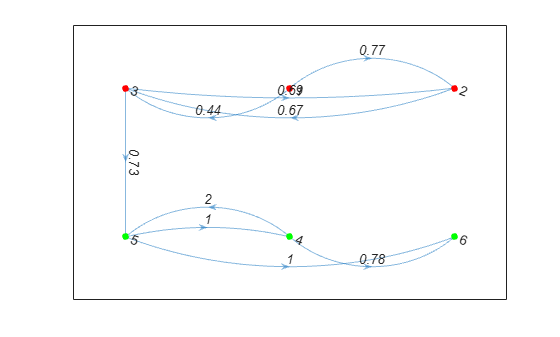## 输入参数

`'searchtrees'`（默认值）

`'augmentpath'`

`'pushrelabel'`

## 输出参数

• 如果 `s``t` 指定数值节点索引，则 `cs``ct` 也包含节点索引。

• 如果 `s``t` 指定节点名称，则 `cs``ct` 也包含节点名称。

• 如果 `s``t` 指定数值节点索引，则 `cs``ct` 也包含节点索引。

• 如果 `s``t` 指定节点名称，则 `cs``ct` 也包含节点名称。

## 详细信息

### 最小割

`cs``ct` 中的条目指示 `G` 的分别与节点 `s``t` 相关联的节点。`cs``ct` 满足 `numel(cs) + numel(ct) = numnodes(G)`